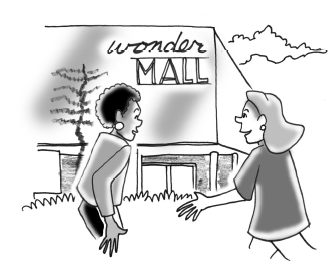### Home > CCAA8 > Chapter 10 Unit 11 > Lesson CCA: 10.2.3 > Problem10-57

10-57.

Marisol and Mimi walked the same distance from their school to a shopping mall. Marisol walked $2$ miles per hour, while Mimi left $1$ hour later and walked $3$ miles per hour. If they reached the mall at the same time, how far from the mall is their school?Since Marisol and Mimi both walked the same distance, write equations for each of their distances.
Be sure to define your variable, $t=$ ...

Let $t=$ amount of time Marisol walked

Marisol: $d=2t$
Mimi: $d=3\left(t-1\right)$

$t=3$ hours
Note: The question asks how far the mall is from their school.